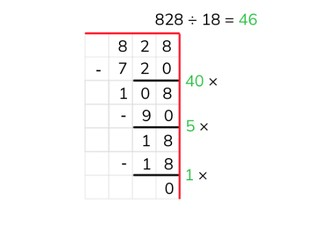Partial quotients division with a number to 1,000

# Partial quotients division with a number to 1,000

Students learn partial quotients division with a number to 1,000.8,000 schools use Gynzy92,000 teachers use Gynzy1,600,000 students use Gynzy

## General

Students learn partial quotients division with a number to 1,000.

## Standards

CCSS.Math.Content.5.NBT.B.5

## Learning objective

Students will be able to use partial quotients to divide a number to 1,000 without remainder.

## Introduction

On the interactive whiteboard are three problems. Have the students solve the problems. Fill in the digits of each of the answers in the boxes. Then use the numbers in the colored boxes to make a new problem and then have the students solve it.

## Instruction

Explain that with partial quotients division you break up the problem into pieces and then solve it like this. You write down the problem and the draw a line underneath it. Then you can use a helping row from the table of the number that you are dividing by. You don't need to write down every problem from the table here. You can also double and halve certain problems, or add a 0 to the end. After this you solve the problem step by step by seeing how many times 28 fits in 588. After every step you write down how many you have left over. If there is nothing left over, then the problem is finished. You then add up all the numbers that tell how many times 28 goes into 588 (40, 5, and 1). Next, have the students solve a few problems using partial quotients on their own. Have them write the problem down on graphing row, and they can also write down a helping row if they need one. Ask various students what steps they used to solve the problems. Encourage the students to take the largest possible steps to solve the problem.

Check whether the students can use partial quotients to divide by asking the following questions:
- Why is it useful to be able to divide using partial quotients?
- What aid can you use when dividing using partial quotients?
- Solve this problem using partial quotients: 299 ÷ 13

## Quiz

The students test their understanding of partial quotients division with a number to 1,000 through ten exercises. In each exercise, the students must solve a division problem using partial quotients. In some of the exercises, the students are given possible answers to choose from. In others, they must provide the answer on their own.

## Closing

You discuss once again with the students that it is important to be able to use partial quotients to divide a number to 1,000, because that is how you can solve problems more easily. Check whether the students know that with partial quotients division they break the problem up into steps and that they can use a helping row to do so. On the interactive whiteboard there are a few problems that are solved using partial quotients. The students must determine what has been done incorrectly in solving each of the problems.

## Teaching tips

When students have difficulty with partial quotients division, you can have them make helping rows of the table of the number that they are dividing by. Have them solve a problem step by step. Look carefully at each of the steps that they make and encourage them to take large steps (not the step 10 × 3 = 30 ten times, but in one step 100 × 3 = 300).

## Instruction materials

Graphing paper

### The online teaching platform for interactive whiteboards and displays in schools

• Save time building lessons

• Manage the classroom more efficiently

• Increase student engagement